ImuCalibration

Theory

Accelerometer and Magnetometer calibration is critical to AHRS performance and can be performed using no special hardware. For the magnetometer, it is very important that the calibration be performed in the fully assembled vehicle, with all systems powered on. This is called hard-iron calibration and will allow us to compensate for any constant parasitic magnetic fields generated by the vehicle. The calibration process consists of finding a set of neutrals and scale factors for each sensor, such as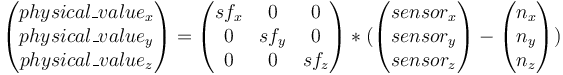$\begin{pmatrix}physical\_value_x\\physical\_value_y\\physical\_value_z\end{pmatrix} = \begin{pmatrix}sf_x&0&0\\0&sf_y&0\\0&0&sf_z\end{pmatrix} * (\begin{pmatrix}sensor_x\\sensor_y\\sensor_z\end{pmatrix}-\begin{pmatrix}n_x\\n_y\\n_z\end{pmatrix})$

The principle of the calibration is the following: an accelerometer, on a vehicle at rest, measures a constant vector (the opposite of gravity) in the earth frame, expressed in the vehicle frame.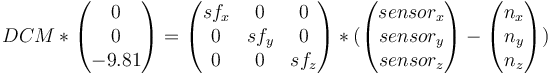$DCM * \begin{pmatrix}0\\0\\-9.81\end{pmatrix} = \begin{pmatrix}sf_x&0&0\\0&sf_y&0\\0&0&sf_z\end{pmatrix} * (\begin{pmatrix}sensor_x\\sensor_y\\sensor_z\end{pmatrix}-\begin{pmatrix}n_x\\n_y\\n_z\end{pmatrix})$

DCM is a rotation matrix that converts between earth frame and body frame. It will change when we change the orientation of the vehicle. Nevertheless, a rotation conserves the norm of a vector. We can thus obtain the following scalar equation that doesn't depend on the vehicle orientation :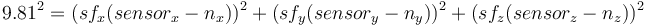$9.81^2 = ( sf_x(sensor_x-n_x) )^2 + (sf_y(sensor_y-n_y) )^2 + (sf_z(sensor_z-n_z) )^2$

We can then record an important number of measurements in different orientations and find the set of scale factors and neutrals giving the norm closest to 9.81

How It Works

• It first makes an initial guess using min and max, i.e. for each axis
• neutral = 0.5 * (max + min)
• sensitivity = 0.5*(max-min)
• It then uses a data fitting algorithm to optimize the initial guess.

Screenshot of Scilab version.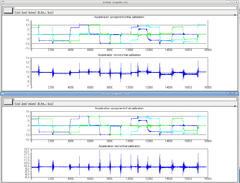Calibration Script Installation

Vital scripts and places:

1. Python script to calibrate the accelerometers and magnetometer. The application can be found in the Paparazzi directory under: sw/tools/calibration/calibrate.py
2. Before you start calibrating clear log directory (PAPARAZZI_HOME/var/logs), it will greatly simplify log file search process.

For the application to work, however, you need additional Python libraries. If you already have the package paparazzi-dev installed, the needed libraries were already installed as dependencies. If this is not the case you need to install python-scipy and python-matplotlib. This can be done via Synaptic Package Manager or via the command-line of Ubuntu.

$sudo apt-get install python-scipy$ sudo apt-get install python-matplotlib

How to Calibrate Your IMU

Basic procedure:

1. Flash the board with your normal AP firmware (if it is not already on it.)
2. Switch to the "raw sensors" telemetry mode via GCS->Settings->Telemetry and launch "server" to record a log.
3. Move your IMU/airframe into different positions to record relevant measurements for each axis.
• It is important that you get the min/max sensor values on each axis!
4. Stop the server so it will write the log file (to var/logs).
5. Run the Python script on it to get your calibration coefficients and add them to your airframe file.
• If you have data from more than one aircraft in your logfile, you will need to specify the aircraft id via the --id option.
It can be found in the Paparazzi Center upper left side. (first comes A/C name, and then id value)

Run the script with the --help to list available options:

sw/tools/calibration/calibrate.py --help

Calibrating the Accelerometers

Since accelerometers are very sensitive to distortions, strapping board to some heavy object like big LiPO battery. It will help keep the board steady while rotating.

To calibrate the accelerometers turn and hold (~10 sec/side) the IMU on all six sides of the cube:

1. upright,
2. inverted,
3. on the nose,
4. on the tail,
5. on the right side,
6. on the left side.

Please note that the IMU axes matter here, and NOT the airframe axes.
So if you have your IMU mounted at an angle, make sure you align the IMU and not the aircraft axes with gravity. Also for the accelerometer calibration the IMU doesn't need to be mounted in the airframe (opposed to the magnetometer calibration).

You can also take some measurements banking 45 degrees.
Try to get a homogeneous distribution of your measurements. It is better to let the aircraft rest while measuring.

Then stop the server so it will write the log file and run the Python script on it to get your calibration coefficients:

sw/tools/calibration/calibrate.py -s ACCEL <var/logs/YY_MM_DD__hh_mm_ss.data>

Calibrating the Magnetometer

First of all it is important to know that all ferromagnetic materials near the mag disort the measurements. So preferably you do the mag calibration with the mag/autopilot mounted in your frame and as far away from metal and magnets as possible.

The most crucial part for the magnetometer calibration: Ideally you would spin it around all axes until you have densely covered the whole sphere with measurements.
Since that is often a bit hard to do with the whole airframe you can also just make sure to really get the min/max on each axis by aligning the magnetometer axes along the local magnetic field vector:

1. Calculate the inclination and declination of the magnetic field where you live that can be looked up here. Just put in zip/country+city/coordinates & date to get proper degrees. See MAG1 figure.
2. Now point the each mag axis in the direction you got from previous step.

It can also be convenient to plot the IMU_MAG_RAW values to see when you get the maximum on each axis.

1. Launch Paparazzi Center->Tools->Realtime_Plotter and Tools->Messages
2. Drag&Drop each axis of the IMU_MAG_RAW message to the Plotter canvas.

After you took all measurements, stop the server so it will write the log file and run the Python script on it to get your calibration coefficients:

sw/tools/calibration/calibrate.py -s MAG <var/logs/YY_MM_DD__hh_mm_ss.data>

This basically does a hard-iron (offset of the sphere) and soft-iron (distortion to an ellipsoid) calibration. You can see the results in Figure MAG2.

The magnetic field changes depending on where you are in the world, because of this you have to set the local magnetic field. Or if you are using the older euler filter recalibrate your magnetometer to fly somewhere else.

Calibrating the Gyroscopes

To calibrate the gyros you need a turntable....
The gyro neutrals (bias) are usually not a problem, since they are set by the AHRS aligner at each startup and some AHRS algorithms continuously estimate the bias during flight.

Finding and Checking Signs

For supported IMUs, the correct default signs are already defined in the code.

If using a new IMU or sign for yours are not in the code yet, here is the way to find them.

We're calibrating everything relative to the IMU frame - Paparazzi has a parameter to define the orientation of the IMU with respect to the body of the vehicle that we'll use later, once you'll have decided of a good mechanical mounting.

Paparazzi uses North East Down (NED) frame, that is positive x is pointing to the front, positive y to the right and positive z down.

Accelerometer:

An accelerometer measures the non gravitational acceleration, that is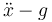$\ddot{x} - g$.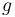$g$ is pointing down, so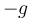$-g$ is pointing up. So stop moving, disregard earth rotation and you'll measure$-g$.

• When your IMU is level you should see x=0 y=0 z=-9.81
• When pitching up -g is aligning with x, so you should see x>0, y=0 and z<0
• When banking left -g is aligning with y, so you should see x=0, y>0 and z<0

Magnetometer:

A magnetometer measures the Earth's magnetic field. In the northern hemisphere, this points north and down and in the Southern hemisphere north and up.

Thus in the northern hemisphere:

• When you align your IMU with the direction of north, you should see x>0, y=0, z>0.
• When pitching the IMU down, the magnetic vector is aligning with x, so x should increase and z should decrease to zero.
• If yawing your IMU to the left, the magnetic vector is aligning with y, so y should be positive, x should decrease to zero and z stay positive.

And in the southern hemisphere:

• When you align your IMU with the direction of north, you should see x>0, y=0, z<0
• When pitching the IMU up, the magnetic vector is aligning with x, so x should increase and z should increase towards zero.
• If yawing your IMU to the left, the magnetic vector is aligning with y, so y should be positive, x should decrease to zero and z stay negative.

Gyrometer:

You need some turntable to calibrate the scale factors of your gyros. For signs, the definition of the frame gives the following properties:

• When rolling right,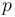$p$ should be positive.
• When pitching up,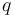$q$ should be positive.
• When yawing to the right,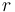$r$ should be positive.

Verification:

Switch to AHRS telemetry mode and look for the fields that are prefixed with imu_

• Bank right should give positive phi
• Pitch up should give positive theta
• Yaw right should give increasing psi
• The value you'll see after letting the IMU rest will end up being the "measure" (that is accelerometer and magnetometer.) If those are wrong, the problem is in the calibration of your sensors.
• The values you get while moving the IMU are influenced by the gyros. If what you see is the value going crazy when you move and then stabilizing to something good after you stop moving, the problem is in your gyros.